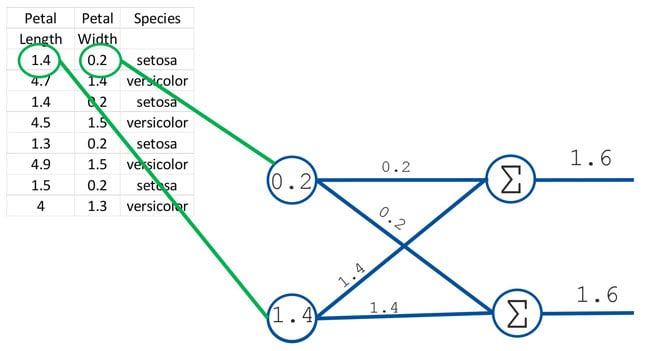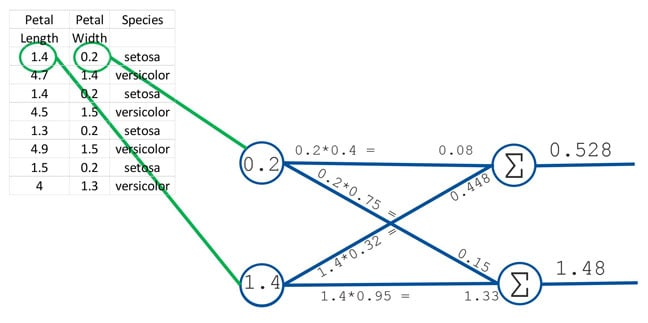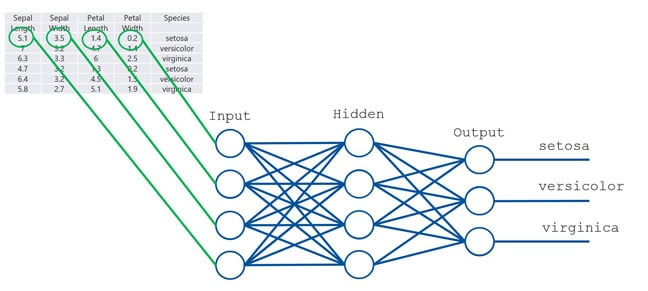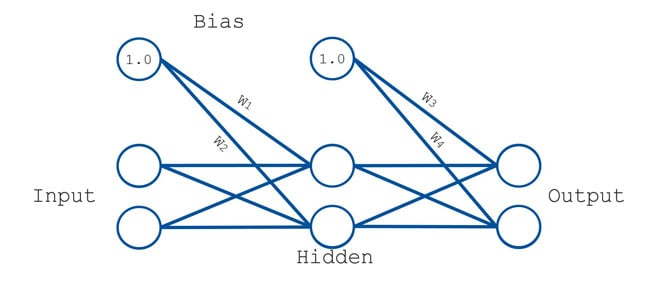# Neural networks: Today, classifying flowers... tomorrow, Skynet maybe

## Here's one I made earlier – now, over to you

If there's a poster child for machine learning, it's neural networks. We gave a practical introduction to the topic here, but this time I'll take a different approach and explain the background to how neural networks, er, work.

To demonstrate this, we'll show how a neural net can be used to classify different species of iris flower (this data set is essentially the "hello world" of machine learning).

One crucial point about neural nets is that, like machine learning in general, they learn the rules for themselves. They are performing induction. Normally, when we write a computer program to solve a problem, we (the humans) work out the rules. Say if you want to work out the species of plant, you ask questions that produce results such as: if the petal length is longer than 3.5mm and so on.

Petal length Petal width Species
1.4 0.2 Setosa
4.7 1.4 Versicolor
1.4 0.2 Setosa
4.5 1.5 Versicolor
1.3 0.2 Setosa
4.9 1.5 Versicolor
1.5 0.2 Setosa
4 1.3 Versicolor

But in machine learning in general, and neural nets in particular, we don't write a program based on our rules, we use the neural net to work out what the rules are and then apply them. So it is a much, much more general purpose tool.

We start with two species of iris – setosa and versicolor. We will design a very simple neural net of just four neurons. Two of them are designed to accept the data (input neurons) and two to output the answer (output neurons).

The data flows from left to right in the diagram and both of the input neurons are connected to both of the output neurons. We set the output neurons to "sum" the values they receive. We arbitrarily decide that we want a large number appearing at the upper output neuron to signify a setosa plant and a large number at the lower one to signify versicolor. Fine. But how can the neural net possibly learn how to output the data like that?

Well, we have to start by training it, meaning that we show it not only the measurements but also the answer. Once we have trained it, we can test it with data where we know the species but we don't tell the neural net; we simply check the answer it gives against what we already know. Once we think it is good enough we can use it to actually do some work for us classifying iris plants of unknown species.

### And we're off...

We take the first set of values from the table (which we know refer to setosa) and input them into the net. The data flows along each connection meaning that, if we were to run the data through, each output neuron would receive and output identical values, which is not what we want.But this is easy to fix. We simply add a numerical weight to each connection and the value that passes through the connection is multiplied by that weight. For the first run of the data these weights are set to random values so it is perfectly possible that the lower output neuron has a higher value.But that's OK because the weights are under the control of the neural net. So it "looks" at the output and then tweaks the weights and tries again. And again. And again until the output value in the upper neuron is higher. Then it feeds in the next set of values (which are from versicolor) and again adjusts the weights, this time trying to produce a high output in the lower output neuron. And it keeps on retrying all the data with different sets of weights until it finds the optimal weight values.

That, in essence, is how a neural net works. However, in practice this particular neural net is very unlikely to yield a good classifier for two main reasons. It is too simple and it isn't optimised.

### Let's ramp it up

We'll solve the first problem by adding more columns of data (and another iris species) and more neurons. This time we will have four input neurons (again, one for each column of data) and three output neurons, one for each species. We will also add a row of what are called "hidden neurons" between the input and output neurons. Here I have added one layer but you can add as many as you like. Each hidden neuron is connected to all the neurons in the layer to its left and to its right. Each connection has a different weight and so, as the data starts to flow from left to right it has far more paths to follow, each of which has a different weight which will change it. There are so many paths that it is much more likely that some combination of weights will be able to produce the correct outputs to identify the species.That's the good news. The bad news is that, while this is a major improvement, it still isn't really practical. That's fine, we can add further improvements to make it work but first it may be helpful to give some of the background to put the importance of these improvements into perspective. Neural nets were proposed in the 1940s but we really struggled to make them work.

Indeed, by 2010 MIT was thinking of dropping neural nets from the teaching syllabus. MIT professor Patrick Winston has said that one of the few compelling reasons for deciding to continue teaching them was that, by doing so, they were insuring that their students didn't think of neural nets for themselves and waste time reinventing them.

However, in 2012 Geoffrey Hinton (emeritus professor of computer science, University of Toronto and Google engineering fellow and also the great, great, grandson of George Boole, the father of differential equations) finally cracked all the practical problems. He made a neural net that blew the competition into the weeds. Since then they have taken the machine learning world by storm. Hinton and his team performed outstanding work but Hinton was also standing on the shoulders of giants, so I'll simply show you the bits we need to add to make neural nets work.

One is that we need to normalise the data. So, for example, you'll notice the sepals are longer than the petals. The larger numbers are liable to overwhelm the smaller so we "normalise" the data – turn all of the data into a set of values between two limits (typically 0 and 1).

### Weights, threshold and bias

This can be done very simply, by multiplying each set of numbers by different scaling factors, or by more complex means. We normalise the weights as well. We usually sum the values when they reach the neuron as already described (although we can choose to do other forms of aggregation) but we then pass the result through a "threshold". This is typically a scalar function and all it does is to ensure that the final output from the neuron is again scaled to give a value between 0 and 1. Finally it is often helpful to add what are called "bias" neurons, which essentially inject numbers into the system. These numbers are also modified by weights.As you can see, the scaling of values throughout the neural nets is very important but we often scale in different ways. A core part of designing effective neural nets is choosing the number and distribution of the neurons and also choosing the correct scaling mechanisms.

Note that, despite these changes, the basic principle of the neural net is still exactly the same. We feed data into the input neurons and it flows through the hidden neurons to the output. It is the weights that cause the value of the data coming out of the output neurons to vary. At first the weights are random but the neural net passes the data through itself many times and it tweaks the weights to try to ensure that the output values are the same for each setosa and different for each of the other species.

Once we have a set of weights that seem to work, we take the data that we held back for testing and feed that through. For this data we know whether it is setosa etc. but we don't tell the neural net so we can test to see how effective it is with data that it hasn't seen before. If it proves to be effective we can start using it to classify iris plants of unknown species.

There are several reasons why we need to add the complexity described above, but the most important is to overcome the problem we have with the number of weights. In our original network we only had four weights. If we imagine that each weight could be set to 100 different values then with just four neurons there are 100,000,000 different combinations of weight values to try.

But four neurons aren't enough; we have to add more. As we do so, the number of possible combinations of weight values rapidly becomes computationally impossible to calculate by brute force. It wasn't until 1974 that Paul Werbos, working at Harvard, came up with the idea of backpropagation. This is a much more efficient algorithm for learning the weights and essentially tunnels under the computational mountain and allows the problem to be solved in a realistic time frame. ®